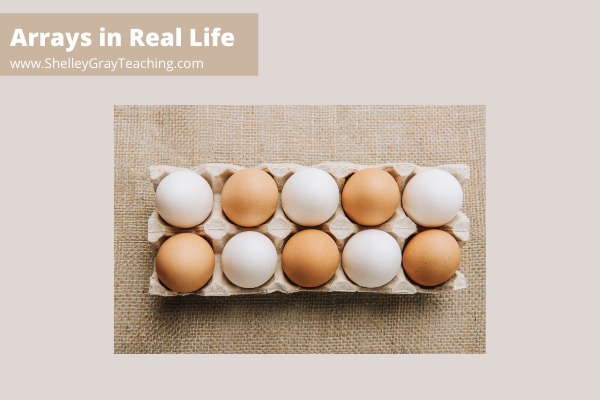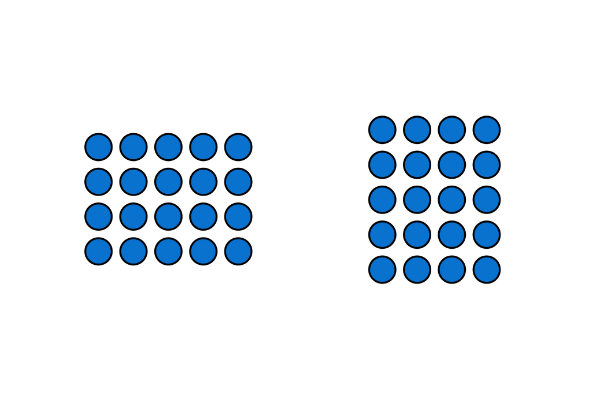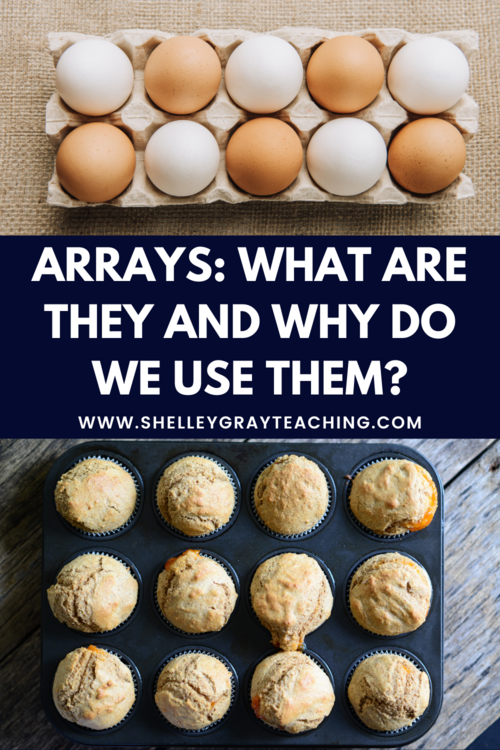# Arrays: What Are They and Why Do We Use Them?An array is a way of arranging objects in groups or columns. In math, we generally see arrays with rows and columns of dots, like this:However, arrays appear in real life too! Take a look at these pictures below:### Why are arrays useful?

Arrays allow us to see a visual representation of repeated addition or multiplication. For example, in the egg carton above, we see 2 rows of 5. We can think of this as 5+5 or 2×5. In the muffin tin, we see 3 groups of 4. We can think of this as 4+4+4 or 3×4.

Arrays also help us see that the order of the factors doesn’t matter when solving a multiplication problem. For example, 3×8 will have the same product as 8×3. To visualize this, we can have our students rotate the arrays. The picture below shows a 4×5 array that has been rotated to show 5×4. This shows us that there are the same number of objects in 5 rows of 4 as there are in 4 rows of 5.### When should arrays be used?

Arrays can be used for addition in the early grades. Students might use blocks, counters, or bingo chips to create arrays. Then a repeated addition sentence can be created. For example, 6 rows of 3 can be written as 3+3+3+3+3+3=18.

Arrays are essential as a tool for multiplication.

We can begin by making equal groups using blocks or counters. Then we can arrange those objects into an array.

### Tips for Using Arrays To Teach Multiplication Concepts:

• Have students work with rotating the array to show the commutative property of multiplication (the order of the factors doesn’t change the product). For example, a 4 by 3 array has the same number of objects as a 3 by 4 array.
• Have students show equal groups using an array. For example, a 4 by 3 array represents 4 groups of 3. This can be written like 4×3.
• Have students break up the array to show the distributive property. For example, a 4×5 array can be broken up into a 3×5 piece and a 1×5 piece. This proves that 4×5 is equal to (3×5)+(1×5).
• We know that our students move through three stages in terms of number – concrete, representational, and finally abstract. Use arrays as the “concrete” part. Then have students represent the array in a drawing (representational). Lastly, have students write an equation to represent the array (abstract). Remember that lots of practice in the concrete and representational stages will help once we expect students to work in the abstract stage!

How do you use arrays in your classroom? I’d love to hear in the comments below!

### PIN FOR LATERThis site uses Akismet to reduce spam. Learn how your comment data is processed.